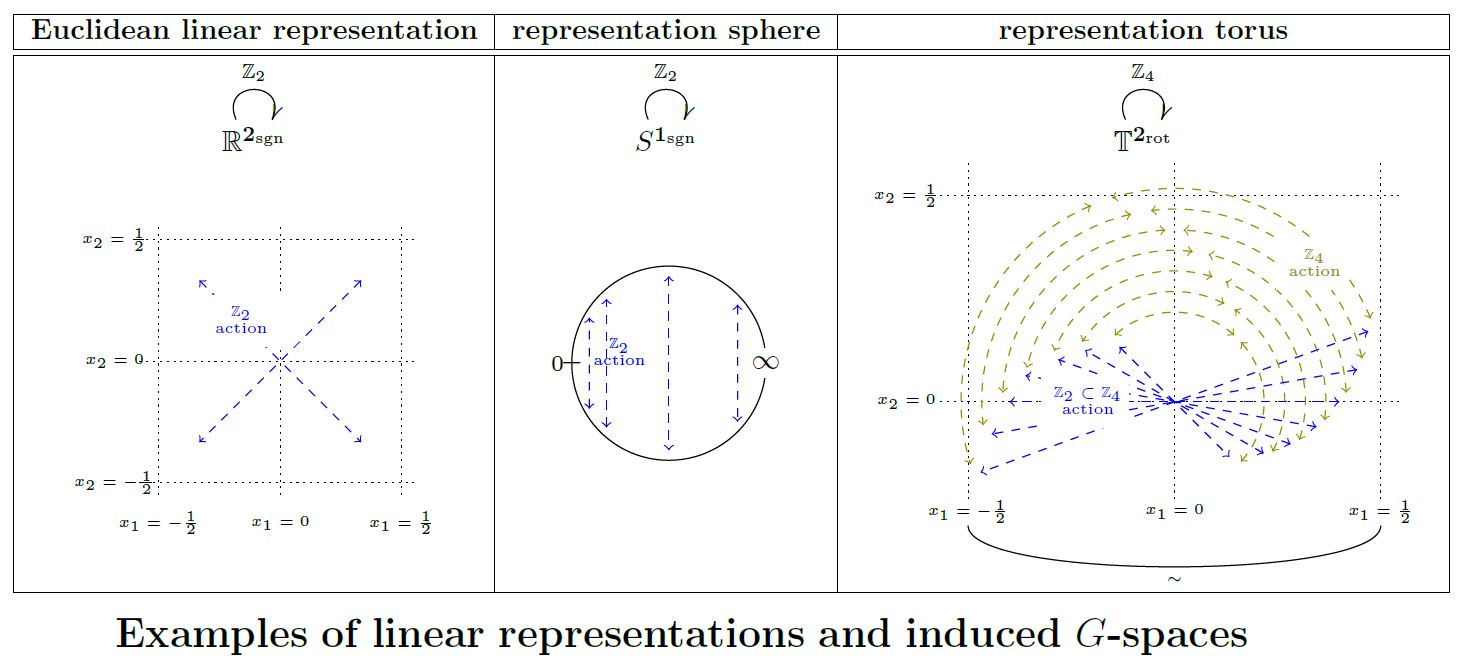Contents

# Contents

## Definition

Let $G$ be a compact Lie group and let $V \in RO(G)$ be an orthogonal $G$-linear representation on a real vector space $V$. Then the underlying Euclidean space $\mathbb{R}^V$ inherits the structure of a topological G-space

We may call this the Euclidean G-space associated with the linear representation $V$.

## Properties

### Relation to representation spheres

The one-point compactification of a Euclidean $G$-space is the corresponding representation sphere:

$\big( \mathbb{R}^V \big)^{cpt} \;\simeq\; S^V \,.$

### Relation to representation tori

Let $V \in RO(G)$ be an orthogonal linear representation of a finite group $G$ on a real vector space $V$.

If $G$ is the point group of a crystallographic group inside the Euclidean group

$N \rtimes G \hookrightarrow Iso(\mathbb{R}^V)$

then the $G$-action on the Euclidean $G$-space $\mathbb{R}^V$ descends to the quotient by the action of the translational normal subgroup lattice $N$ (this Prop.). The resulting $G$-space is an n-torus with $G$-action, which might be called the representation torus of $V$graphics grabbed from SS 19

### Equivariant configurations in Euclidean $G$-spaces

(…)

Discussion of equivariant configuration spaces of points in Euclidean $G$-spaces: